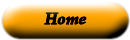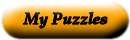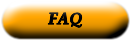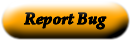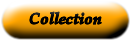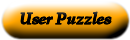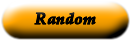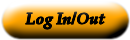### MATH VOCAB (PINK)

RENEE EARL

5TH GRADE VOCAB MOSTLY GEOM AND DATA TERMS

 1 vertex _____ When a set of data is in numerical order, this is the middle 2 Acute triangle _____ The smallest value in a set of data 3 Equilateral triangle _____ A triangle in which all three sides are different 4 isosceles triangle _____ In a set of numbers, the mode is the number that appears the most 5 obtuse triangle _____ A triangle with one obtuse angle. 6 scalene triangle _____ The average 7 divide _____ The number in a division problem that is being divided. 8 dividend _____ A triangle which has at least 2 sides and 2 angles 9 chance _____ A number multiplied by itself 10 estimate _____ The possibility of an event happening. 11 maximum _____ A triangle with 3 equal sides and 3 equal angles 12 mean _____ How many times the base is multiplied by itself 13 median _____ Rounding a number to about the same value 14 minimum _____ To separate a number of items into groups of the same size. 15 mode _____ Triangle in which all 3 angles are acute. 16 range _____ The largest value in a set of data 17 square number _____ The point in a polygon where two lines intersect (the 18 exponent _____ The difference between the maximum and the minimum

Use the "Printable HTML" button to get a clean page, in either HTML or PDF, that you can use your browser's print button to print. This page won't have buttons or ads, just your puzzle. The PDF format allows the web site to know how large a printer page is, and the fonts are scaled to fill the page. The PDF takes awhile to generate. Don't panic!Web armoredpenguin.com